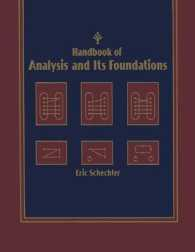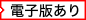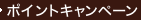••### Handbook of Analysis and Its Foundations

• ただいまウェブストアではご注文を受け付けておりません。 ⇒古書を探す
• 製本 Hardcover:ハードカバー版／ページ数 883 p.
• 言語 ENG
• 商品コード 9780126227604
• DDC分類 515

### 基本説明

Provides an introduction set theory and mathematical logic; algebra; topology; normed spaces; integration theory; topological vector spaces; and differential equations.

### Full Description

Handbook of Analysis and Its Foundations is a self-contained and unified handbook on mathematical analysis and its foundations. Intended as a self-study guide for advanced undergraduates and beginning graduatestudents in mathematics and a reference for more advanced mathematicians, this highly readable book provides broader coverage than competing texts in the area. Handbook of Analysis and Its Foundations provides an introduction to a wide range of topics, including: algebra; topology; normed spaces; integration theory; topological vector spaces; and differential equations. The author effectively demonstrates the relationships between these topics and includes a few chapters on set theory and logic to explain the lack of examples for classical pathological objects whose existence proofs are not constructive. More complete than any other book on the subject, students will find this to be an invaluable handbook. For more information on this book, see http://math.vanderbilt.edu/

### Contents

Sets and orderingssups and infs; sets of sets - filters topologies; constructivism and choice; nets and convergences. Algebra: elementary algebraic systems; concrete categories; the real numbers; linearity; convexity; boolean algebras; logic and intangibles. Topology and uniformity: toplogical spaces separation and regularity axioms; compactness; uniform spaces; metric and uniform completeness; Baire theory; positive measure and integration. Topological vector spaces: norms; normed operators; generalized Riemann integrals; Frechet derivatives; metrization of groups and vector spaces; barrels and other features of TVSs; duality and weak compactness; vector measures; initial value problems.